# Representations: morphisms and induction functor

### Schur's lemma and Frobenius reciprocity for induction and restriction

created: 17 Sep 2019; modified: 13 Nov 2019; status: finished

# Morphisms

The standard way to introduce a representation of a group $G$ is as a pair $(\rho,V)$ of a vector space $V$ and a group homomorphism $\rho: G \to \GL(V)$. That is, for $g_1,g_2 \in G$, $\rho(g_1)$ and $\rho(g_2)$ are invertible matrices over a vector space $V$ such that the group structure is respected: $\rho(g_1)\rho(g_2) = \rho(g_1 g_2)$. Group homomorphisms are examples of ubiquitous structure-preserving maps or morphisms between mathematical objects. Morphisms for sets are arbitrary functions (total i.e. defined for all inputs) between sets. For vector spaces, we have linear operators. Of course, not only algebraic objects have morphisms. For example, continuous maps and smooth maps are morphisms, respectively, for topological spaces and smooth manifolds. (In particular, homeomorphisms are isomorphisms between topological spaces, and diffeomorphisms are isomorphisms between smooth manifolds.)

Once we have objects and morphisms, we have a category. Formally, a category $\mathcal{C}$ is a class 1 of objects and morphisms between them such that there is an identity morphism $1\circ f = f\circ 1 = f$ and the composition of morphisms is associative: $(f \circ g) \circ h = f \circ (g \circ h)$. It should be emphasized that all the information contained in the definition is about morphisms, whereas the objects can be anything. (Perhaps the lesson here is that morphisms are as important, if not more, than the objects we want to study.) What would be the morphisms in a category of $G$-representations? They are $G$-equivariant maps or intertwiners. Let $(\rho,V)$ and $(\sigma,W)$ be $G$-representations. A linear map $\varphi:V\to W$ is $G$-equivariant if it does not matter if one applies a transformation $g$ on the vector space first or $\varphi$ first. That is, $\varphi\circ\rho(g) = \sigma(g)\circ\varphi$ for all $g \in G$. Pictorially, we say that the following diagram “commutes”.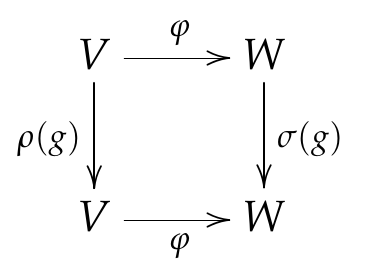When $\rho=\sigma$, this just says that $\varphi$ commutes with $\rho(g)$ for all $g \in G$. This is a pretty natural definition (and should be formalizable as a natural transformation in category theory) but can be made more obvious by thinking of $G$-representations as $k[G]$-modules.

## In terms of modules

The notion of a module generalizes that of a vector space by extending multiplication by scalars to elements of a ring (such as matrices). Given a ring $R$ with an identity, a (left) $R$-module is an abelian group $M$ representing “vector addition”, and “scalar multiplication” $R \times M \to M$ a ring homomorphism from $R$ to the endomorphism ring $\End(M)$ that also respects the group addition; in equations, \begin{align} 1m &= m, \\ (r+s)m &= rm + sm, \\ (rs)m &= r(sm), \\ r(m+n) &= rm + rn, \end{align} for all $r,s \in R$ and $m,n \in M$. A right $R$-module or even a left-right $(S,R)$ bi-module is similarly defined but with the R-multiplication from the right and $S$-multiplication from the left. (Left and right $R$-modules may not coincide.) Now the ring $k[G]$ is the matrix ring formally spanned by $g \in G$ over a field $k$ with the convolution product * inherited from the group multiplication: \begin{align} \left(\sum_{g_1}f_{g_1} g_1 \right) * \left(\sum_{g_2}h_{g_2} g_2 \right) = \sum_{g_1,g} f_{g_1} h_{g_1^{-1}g} g. \end{align} In fact, $k[G]$ is not just a ring but also an algebra (because it is a vector space) called the group algebra. Given a $G$-representation, the linear span of $\rho(g)$ gives a $k[G]$-module. Conversely, given a $k[G]$-module, there is a vector space $V$ over $k$ (because $k\id \in k[G]$), on which the $G$-action gives a group homomorphism $G \to \GL(V)$, that is, a representation. In this language, intertwiners are linear maps that commute with multiplication by scalars in $k[G]$. In other words, they are $k[G]$-linear maps, generalizing the fact that linear operators (all of which commute with the scalars) are morphisms in the category of vector spaces.

## Schur’s lemma

Intertwiners between irreps are completely characterized by Schur’s lemma, a very basic (easily proved using only a little linear algebra) but powerful result (and obviously what inspired the title of this blog). Denote the space of intertwiners between $G$-representations $V$ and $W$ by $\Hom_G(V,W)$ (and $\End_G(V)$ if $V=W$).

Lemma 1. (Schur’s lemma)

• (Burnside) Every intertwiner between irreps is either an isomorphism or zero.
• (Schur) For $k$ algebraically closed, every intertwiner is of the form $k\id$. That is, $\End_G(V) = k$. 2

The lemma can also be summarized as follows: when $k$ is algebraically closed, intertwiners between irreps behave like the Kronecker delta \begin{align} \dim\Hom_G(V,W) = \begin{cases} 1, V \cong W, \\ 0, V \;\unicode{0x2246}\; W. \end{cases} \end{align}

## The meaning of tensor operator

I claim that one does not really understand the concept of tensor operators in physics until one understands intertwiners. Why? Because tensor operators are, by definition, intertwiners. This can be seen by unpacking the seemingly circular definition of tensor operators as “operators that act like tensors”. For concreteness, let us think about one of the simplest non-trivial cases of a vector operator. Let $(R,\R^3)$ be the defining representation of $\SO(3)$ and $\mathbf{r} \in \R^3$. A vector operator $T(\mathbf{r})$ has components $T_j \coloneqq T(\mathbf{r}_j):V\to W, j=1,2,3$ that are linear maps between irreps $(\rho,V),(\sigma,W)$ of $\SO(3)$ (that is, $T_j \in\Hom(V,W) = V^* \otimes W$) such that the $G$-action on $\Hom(V,W)$ (a superoperator $\sigma\dgg(R) \odot \rho(R)$ where $\odot$ is a placeholder for an argument) induces a rotation of $\mathbf{r}$: 3 \begin{align} \sigma\dgg(R)T(\mathbf{r}_j)\rho(R) = R_j{}^k T(\mathbf{r}_k). \end{align} Writing this in the coördinate-independent form \begin{align} \sigma\dgg(R)T(\mathbf{r})\rho(R) = T(R\mathbf{r}), \end{align} one can see that $T$ is nothing but a linear map that makes the following diagram commutes!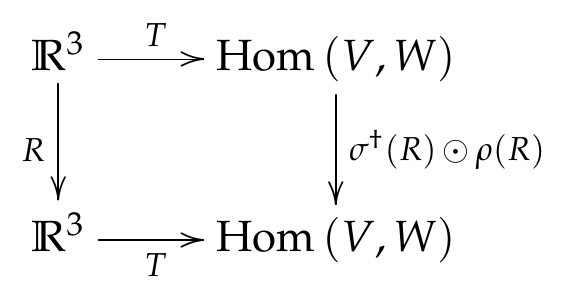By the first part of Schur’s lemma, $T$ is an isomorphism between $(R,\R^3)$ and its copy within $\Hom(V,W)$ if it exists. 4 This justifies labeling the components of the image $T_j$ the same way we label the components of a vector $\mathbf{r} \in \R^3$. More generally, $\R^3$ can be replaced by any irrep $U$ of $\SO(3)$.

Does anyone remember what the celebrated Wigner-Eckart theorem says in the form usually taught in a quantum mechanics class? Definitely not me. But by recognizing tensor operators as intertwiners, the theorem is just Schur’s lemma in disguise, specialized to intertwiners between $U$ and $\Hom(V,W)$; the basis-independence of the “reduced matrix element” $\av{j',m'||T_k||j,m}$ is just the statement that the tensor operator is proportional to the identity $T = c\id$ (because it commutes with every $G$-action because it is $G$-equivariant) in the block isomorphic to the irrep $U$.

# Induced representations

Given a representation of $G$, we can always restrict it to a representation of a subgroup $H \subset G$. What about the inverse process of constructing a $G$-representation out of an arbitrary $H$-representation? The language of categories and modules will prove useful here. Restriction is a functor from the category of $G$-representations to the category of $H$-representations. Given categories $\mathcal C$ and $\mathcal D$, a (covariant) functor $\mathcal{F}:\mathcal C\to \mathcal D$ assigns to every object in $\mathcal C$ an object in $\mathcal D$ and to every morphism in $\mathcal C$ a morphism in $\mathcal D$: \begin{align} \mathcal{F}:\Hom_{\mathcal C}(A,B) \to \Hom_{\mathcal D}(\mathcal{F}(A),\mathcal{F}(B)), \end{align} where $A,B\in\mathcal C$, such that the identity and the composition is preserved: \begin{align} \mathcal{F}(1_{\mathcal C}) &= 1_{\mathcal D}, \\ \mathcal{F}(f\circ g) &= \mathcal{F}(f) \circ \mathcal{F}(g). \end{align} Restriction belongs to a simple kind of functors, forgetful functors that simply forget information, in this case the structure of $G$. Restriction therefore cannot have a strict inverse. An insight from category theory is that we can construct the induction functor as a kind of “weak inverse” to the restriction functor called an adjoint. More precisely, induction should be define as a left adjoint (because $\mathrm{Ind}$ is in the left slot) \begin{align} \Hom_G(\mathrm{Ind}_L\,V,W) \cong \Hom_H(V,\mathrm{Res}\,W) \end{align} or a right adjoint \begin{align} \Hom_G(V,\mathrm{Ind}_R\,W) \cong \Hom_H(\mathrm{Res}\,V,W) \end{align} to the restriction functor $\mathrm{Res}$. 5 These canonical isomorphisms (14,15) of intertwiner space are known as Frobenius reciprocity. (We will see that the distinction between left and right adjoint comes precisely from the choice of thinking of $k[G]$ either as a $(k[G],k[H])$-bi-module or as a $(k[H],k[G])$-bi-module.)

The point that induction is defined so that it satisfies Frobenius reciprocity might be lost if we give a definition of an induced representation first and then prove the adjunction. Not only will it be unclear why the definition given is the right one, there are also inequivalent definitions of induced representation: \begin{align} \mathrm{Ind} W &= \bigoplus_{k \in G/H} kW && \text{(Induction for finite groups)}, \\ \mathrm{Ind} W &= \{f:G\to W|f(gh)=\sigma(h^{-1})f(g) \} && \text{(Induction for Lie groups)}, \\ \mathrm{Ind} W &= \{f:G\to W|f(gh)=\sigma(h^{-1})f(g), f \textrm{ has compact support on } G/H \} && \text{(Compact induction for Lie groups)}, \end{align} to give a few. The functorial viewpoint makes it clear that Frobenius reciprocity is not merely a property but the raison d’être of induced representation.

## Application: Multiplicity

Before we delve into what Frobenius reciprocity really means, let us take for granted the definition of the induced representation for finite groups and think about what they are. Inspecting the simplest case when $H$ is just the identity element would tell you that the induced representation $\mathrm{Ind} 1 = \bigoplus_{g}g\ket{1}$ where $\ket{1}$ is the (one-dimensional) trivial irrep, is one in which every vector can be written as a formal linear combination of group elements of $G$ and $G$ acts by group multiplication $g\ket{g'} = \ket{gg'}$ ($g\ket{1}=\ket{g}$). This is nothing but the represention on the group algebra $k[G]$, the (left) regular representation. Along the same line of thinking, the representation induced from the trivial representation of a subgroup $H$ is the coset representation.

Let $\hat{G}$ be the collection of all inequivalent irreps of $G$. Every completely reducible representation (by definition) decomposes into the orthogonal direct sum of irreps $V_{\lambda}$ \begin{align} V &\stackrel{G}{\simeq} \bigoplus_{\lambda \in \hat{G}} \bigoplus^{n_{\lambda}} V_{\lambda}, \end{align} each with (possibly zero) multiplicity $n_{\lambda}$. An important special is when the left hand side is a tensor product of irreps, then (19) is the Clebsch-Gordan decomposition, the multiplicities in which when $G$ is a unitary group $\U(d)$ are known as the Littlewood-Richardson coefficients.

We are going to rewrite (18) so that there is no sum over multiplicities using Schur’s lemma magic. \begin{align} V & \stackrel{G}{\simeq} \bigoplus_{\lambda \in \hat{G}} V_{\lambda} \otimes \mathrm{Hom}_G (V_{\lambda},V) \end{align} because \begin{align} \mathrm{Hom}_G (V_{\lambda},V) &\simeq \bigoplus^{n_{\lambda}} \mathrm{Hom}_G ( V_{\lambda}, V_{\lambda} ) \simeq \mathbb{C}^{n_{\lambda}}. \end{align} (19) is called an isotypic decomposition of a representation where each term in the summand is an isotype. Now $n_{\lambda}$ is the dimension of the multiplicity space $\mathrm{Hom}_G (V_{\lambda},V)$ which is exactly what shows up in Frobenius reciprocity! In particular, \begin{align} \dim \Hom_G(\mathrm{Ind}_L\,V,W) = \dim \Hom_H(V,\mathrm{Res}\,W) \end{align} says that the number of times a $G$-irrep $W$ appears in the representation $\mathrm{Ind}V$ induced from $V$ is the same as the number of times the restriction $\mathrm{Res}W$ to $H$ appears in an $H$-irrep $V$. 6

When the representation is induced from the trivial subgroup, we immediately obtain the well-known result (typically shown via character theory) that every irrep of a finite group $G$ appears in the regular representation as many times as its dimension and in particular $\dim G = \sum_{\lambda\in\hat{G}} (\dim V_{\lambda})^2.$ But we can induce from subgroups beyond the trivial subgroup and representations beyond the trivial representation. Induced representations have applications in the classification of representations of the Lorentz group by Wigner little groups, in Harish-Chandra’s parabolic induction to find representations of reductive groups, or to the theory of spherical representations (or more algebro-geometrically spherical varieties).

### Example: The simplest adjoint functor

What does an adjoint functor look like in a category with only one morphism? Such a morphism $x\to y$ can be though of as a comparison $x\le y$, a preorder if you will 7. (I learned this example from John Baez’ online Applied Category Theory Course. See also Qiaochu Yuan’s answer] from Math Stack Exchange) A functor between two preorders $\mathcal C$ and $\mathcal D$ is a monotone function if it is order-preserving: $a \le_{\mathcal C} b \implies f(a) \le_{\mathcal D} f(b).$ A pair of monotone functions $f$ and $g$ is an adjoint pair if there is a one-to-one correspondence $f(a) \le_{\mathcal D} x \iff a \le_{\mathcal C} g(x).$ Suppose, for instance, that $\mathcal A = \mathcal B = \mathbb{N}$, the natural numbers. The monotone function $x \to 2x$ has no strict inverse because $y/2$ may not be an integer, but it does have right and left adjoints: the ceiling $\lceil x/2 \rceil$ and the floor $\lfloor x/2 \rfloor$ respectively. (Verify this.) Adjunction generalizes this one-to-one correspondence.

### Example: Free functor

Many useful constructions, like those of induced representations, are adjoint functors of a forgetful functor. The free functor $\mathcal F:$ from the category of all sets to the category of all groups sends any set to its free group, the group of all “words”, non-commutative product constructed from the alphabet in $S$ and their inverses i.e. $S$ generates $\mathcal F(S)$ without any constraint. The free functor is defined by the universal property 8 that giving a function $f$ from $S$ to (the underlying set of) an arbitrary group $G$ is equivalent to giving a unique group homomorphism $\varphi$ from the free group $\mathcal F(S)$ to $G$. For example, the empty set gives the trivial group with one element. (The empty word is the identity.) In other words, $\mathcal F(S)$ can simulate any interaction between $S$ and an arbitrary group $G$ and does so optimally (the uniqueness of $\varphi$).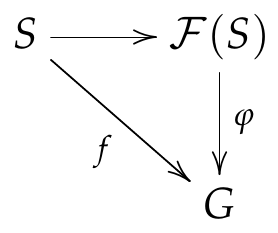The free functor $\mathcal F$ should be an adjoint of the forgetful functor $\mathcal R$ that maps a group to its underlying set. This is because every group homomorphism $\varphi :F(S) \to G$ is completely determined if we know what it does to each generator of $\mathcal F(S)$. Since the generators are just elements of $S$, what $\varphi$ really acts on is the underlying set of the group. Thus, we have the following commutative diagram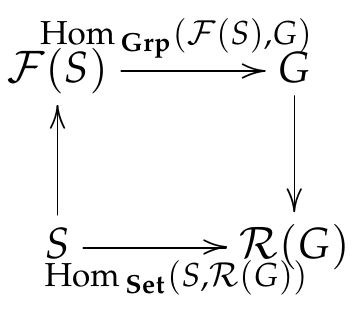which says that the free functor is a left adjoint of the forgetful functor: \begin{align} \Hom_{\mathbf{Grp}}(\mathcal F(S),G) \cong \Hom_{\mathbf{Set}}(S,\mathcal R(G)). \end{align}

Beware that having an adjoint on one side does not imply having the adjoint on the other side, as the forgetful functor from Grp to Set illustrates. Having the right adjoint would imply the following commutative diagram.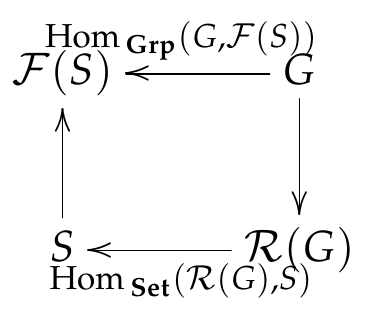But this is impossible when $S=\emptyset$ because there is no (total) function to an empty set, whereas there is always a (single, unique) group homomorphism to $\mathcal F(\emptyset)$ the trivial group with one element (which is indeed the terminal object in Grp).

Having seen some examples of functors, let us go back to our original goal of making sense of induction. In the language of modules, induction corresponds to an extension of scalars, similar to how a real vector space $V$ can be complexified to $V^{\C} = V \otimes_{\R} \C$. We begin by looking for an adjunction involving tensor products.

A hint comes from a trivial identity given three finite-dimensional vector spaces $M,N,P$ \begin{align} \Hom (P\otimes M,N) = \Hom (M,\Hom(P,N)) \end{align} since they both express $P^* \otimes M^* \otimes N$ but without using the dual. This makes the tensoring with $P$ and the “hom” map $\Hom(P,\odot)$ look like an adjoint pair, the tensoring being the left adjoint and hom being the right adjoint. Interestingly, this adjunction continues to hold when the vector spaces, which are $k$-modules, are replaced by general modules. Then it is called the tensor-hom adjunction \begin{align} \Hom_S (P\otimes_R M,N) = \Hom_R (M,\Hom_S(P,N)). \end{align} To unpack its meaning, we have to properly define what $M,N,P$ and these tensor products between modules are.

The tensor product $M \otimes_R N$ of a right $R$-module $M$ and a left-$R$-module $N$ is the vector space $M \otimes N$ quotient by the relation $mr \otimes n - m \otimes rn = 0$ for every $r \in R$. (“$\otimes$ commutes with $R$.”) 9 With a $(S,R)$-bi-module $P$ at our disposal, we can turn an $R$-module into an $S$-module and vice versa. Let $M$ be a left $R$-module and $N$ be a left $S$-module.

• $P \otimes_R M$ is a left $S$-module.
• $\Hom_S (P,N)$ is a left $R$-module.

$\Hom_S (P,N)$ makes sense because both $P$ and $N$ are left $S$-modules. The obvious $R$-action $r\varphi(p) = \varphi(pr)$ turns $\Hom_S (P,N)$ into a left $R$-module. One can verify that the action satisfies the axioms for modules, for example, \begin{align} (r_1 r_2) \varphi(p) = \varphi(p (r_1 r_2)) = r_2 \varphi(p r_1) = r_1 (r_2 \varphi(r)). \end{align}

Now we can give a proof of the tensor-Hom adjunction \begin{align} \Hom_S (P\otimes_R M,N) = \Hom_R (M,\Hom_S(P,N)), \end{align} the only thing complicated about which is the bookkeeping.

Proof.

$\blacksquare$ In one direction, given an $S$-module homomorphism $\varphi$ from $P \otimes_R M$ to $N$, we want to define a corresponding $R$-module homomorphism from $M$ to $\Hom_S (P,N)$. By linearity, $\varphi$ is determined by its values on all rank-one tensors \begin{align} \varphi(p \otimes_R m) \in n. \end{align} So let us define \begin{align} \psi_m(\odot) \coloneqq \varphi(\odot \otimes_R m). \end{align} The $S$-linearity of $\psi_m$ follows immediately from the $S$-linearity of $\varphi$. The $R$-linearity of $\psi$ is not hard to show either: \begin{align} \psi_{rm_1 + m_2}(p) = \varphi(p \otimes_R (rm_1 + m_2)) = \psi_{m_1}(pr) + \psi_{m_2}(p) = r\psi_{m_1}(p) + \psi_{m_2}(p). \end{align} (The last equality is just the previously defined left $R$-action on $\Hom_S (P,N)$).

Conversely, given an $R$-module homomorphism $\psi$ from $M$ to $\Hom_S (P,N)$, define \begin{align} \varphi(\odot_1 \otimes_R \odot_2) \coloneqq \psi_{\odot_2} (\odot_1{}_{}) \end{align} and extend by linearity. $\varphi$ can be verified to be $B$-linear. Finally, since the construction of $\psi$ from $\varphi$ and vice versa is an inverse of each other, the tensor-hom adjunction is established. $\Box$

### (Co-)extension of scalars

I claim that the restriction of scalars from $R$ to a subring $S\subset R$ have both left and right adjoints. Again, $M$ is a left $R$-module and $N$ is a left $S$-module.

• Thinking of $R$ as an $(R,S)$-bi-module, $\mathrm{Res} N \coloneqq \Hom_R (R,N)$ gives a left $S$-module. Then by the tensor-hom adjunction $\Hom_S (N,\Hom_R (R,M)) \cong \Hom_R (R \otimes_S N,M),$

we have that the left adjoint of restriction is $\mathrm{Ind}_L N = R \otimes_S N$, often called the extension of scalars.

• Thinking of $R$ as a $(S,R)$-bi-module, $\mathrm{Res} M \coloneqq R \otimes_R M$ gives a left $S$-module. Then by the tensor-hom adjunction $\Hom_S (R \otimes_R M,N) \cong \Hom_R (M,\Hom_S (R,N)),$

we have that the right adjoint of restriction is $\mathrm{Ind}_R N = \mathrm{Hom}_S (R,N)$ called the co-extension of scalars to distinguish it from the left adjoint.

Setting $R = k[G]$ and $S = k[H]$ gives both versions of Frobenius reciprocity (12,13): \begin{align} \Hom_G(k[G]\otimes_{k[H]} V,W) &\cong \Hom_H(V,\mathrm{Res}\,W),\\ \Hom_G(V,\Hom_{k[H]}(k[G],W)) &\cong \Hom_H(\mathrm{Res}\,V,W). \end{align} We may call the former induced representation of $V$ and the latter co-induced representation of $W$. Clearly, $k[G]\otimes_{k[H]} V$ in (37) is the same as $\mathrm{Ind}_L W = \bigoplus_{k\in G/H} kW$.

There is much more that can be said about induced representations. (How the right and left adjoints for finite groups are isomorphic. How they are in general not isomorphic for Lie groups. The geometric interpretation of adjoint representation as a homogeneous vector bundle.) But those would be topics for another day.

1. The word “class” cannot be replaced by “set”. For example, there is no set of all sets (Russell’s paradox).

2. The assumption that $k$ is algebraically closed is necessary. Consider a representation of $\Z_4$ on $\R^2$ as discrete rotations with the generator $\rho(1) = \begin{pmatrix} 0 & -1 \\ 1 & 0 \end{pmatrix}$. It is irreducible because $\rho(1)$ cannot be diagonalized over $\R$. But every $\rho(g)$ commutes with matrices of the form $a\id + b\rho(1)$ for some $a,b \in \R$, a two-dimensional real vector space.

3. We think of $R$ as both a rotation matrix (the defining representation of $\SO(3)$) and an abstract group element. We also assume the Einstein summation convention.

4. Why can’t there be more than one copy of $(R,\R^3)$ in $\Hom(V,W)$? Because every tensor product $V\otimes W$ of irreducible $\SO(3)$-representations decomposes without multiplicity. Wigner gave a characterization of some of these so-called simply reducible groups which was later generalized by Mackey.

5. The name “adjoint” comes from the similarity to how the adjoint of a linear operator in an inner product space is defined: $\langle x,Ay\rangle = \langle A\dgg x,y\rangle$.

6. As a counterexample to the statement when $V$ is reducible, take $V$ to be multiple copies of the trivial irrep of an abelian group $G$ and take $W$ to be the trivial irrep of some subgroup. $W$ is contained in $\mathrm{Res}V$ but $V$ is not contained in $\mathrm{Ind}W = k[G]$ since the regular representation of an abelian group contains only one copy of each irrep.

7. A partial order is a preorder that is also “antisymmetric”: $a \le b$ and $b \le a$ implies $a=b$. Also for the curious, the product in this category is the infimum and the coproduct is the supremum.

8. I recommend reading this blog post by Jeremy Kun. Excerpt: “There are only two universal properties and they are that of being initial and final.”

9. A tensor product of two nonzero modules can turn out to be zero. For example, $\Z/2\Z \otimes_{\Z} \Z/3\Z = 0$ because $1m\otimes_{\Z} n = (3-2)m\otimes_{\Z} n = m\otimes_{\Z} 3n - 2m\otimes_{\Z} n = 0$ vanishes identically for any $m \in \Z/2\Z$ and $n \in \Z/3\Z$. More generally, the same conclusion can be reached for $\Z/a\Z \otimes_{\Z} \Z/b\Z$ when $a$ and $b$ are coprime since we can always find integers $x$ and $y$ such that $ax+by=1$.

Archieves by date, by category, by tag.